# Study of Models Using One or Two Exponentials to Simulate the Characteristic Current-voltage of Silicon Solar Cells

Study of Models Using One or Two Exponentials to Simulate the Characteristic Current-voltage of Silicon Solar Cells

Inchirah Sari-Ali* Boumédiène Benyoucef  Bachir Chikh-Bled  Younes Menni  Ali J. Chamkha  Giulio Lorenzini

Unit of Research on Materials and Renewable Energies, Department of Physics, Faculty of Sciences, Abou Bekr Belkaid University, B.P. 119, 13000, Tlemcen, Algeria

Mechanical Engineering Department, Prince Sultan Endowment for Energy and Environment, Prince Mohammad Bin Fahd University, Al-Khobar 31952, Saudi Arabia

Department of Engineering and Architecture, University of Parma, Parco Area delle Scienze, 181/A, Parma 43124, Italy

Corresponding Author Email:
chirakurmer@gmail.com
Page:
285-289
|
DOI:
https://doi.org/10.18280/ejee.210304
2 March 2019
|
Accepted:
24 April 2019
|
Published:
15 August 2019
| Citation

OPEN ACCESS

Abstract:

The production of electricity based on the conversion of the sunlight by photocells crystalline silicon is the most way using on technological and industrial plan. As a consequence, the development of the applications of energy production requires cells with high efficiency and low cost. We propose two models using either one or two exponentials allowing to simulate the characteristic current-voltage of a solar cell. The goal of our work is to present a comparative study between the model theoretical and experimental aiming at the improvement of the solar cell efficiency. We also determine the parameters of the solar cell from the current-voltage curve. Additionally, we provide a justification for using the two exponential models for improving the cell efficiency. The model with two exponential allowing to investigate the phenomena of recombination in zone of diffusion and space charge in the areas quasi-neutrals of the transmitter and the base. This study underlines the insufficiency of the model to exponential generally used by showing that it leads to the design of the ideal solar cells whereas structures characterized by a factor of quality greater outcome with high efficiency.

Keywords:

solar cells with high efficiency and low cost, solar cell efficiency, characteristic current-voltage of solar cell, production of electricity, silicon

1. Introduction

A solar cell is an electronic device that produces electricity when light falls on it. The light is absorbed and the cell produces dc voltage and/or current. The device has a positive and a negative contact between which the voltage is generated and through which the current can flow. Solar cells have no moving parts. Effectively they take light energy and convert it into electrical energy in an electrical circuit, Figure 1.

## f1.jpgFigure 1. The photovoltaic effect in a solar cell

Under illumination, a photocell behaves as a generator of power; the characteristic I-V is described for an illumination and a temperature given by the implicit equation :

I=h(I,V)   (1)

With:

$I={{I}_{ph}}-\frac{V+{{R}_{s}}I}{{{R}_{sh}}}-{{I}_{01}}\left[ \exp \left( \frac{q\left( V+{{R}_{s}}I \right)}{KT} \right)-1 \right]\,\,-{{I}_{02}}\left[ \exp \left( \frac{q\left( V+{{R}_{s}}I \right)}{nKT} \right)-1 \right]\ \ \$   (2)

This equation implicit expression for the IV characteristic according to the double exponential model for solar cells.

Or photo generated current (Iph), two diode saturation current (I01 and I02), the series resistance Rs, and the shunt resistance Rsh.

This equation implicit expression for the IV characteristic according to the double exponential model for solar cells.

Or photo generated current (Iph), two diode saturation current (I01 and I02), the series resistance Rs, and the shunt resistance Rsh.

A- The single exponential model (SEM):

Experimentally this model is corrected by the introduction of the factor the quality n, the characteristic courant-tension of solar cell is this described by the following equation, with I02 = 0:

$I={{I}_{ph}}-\frac{V+{{R}_{s}}I}{{{R}_{sh}}}-{{I}_{01}}\left[ \exp \left( \frac{q\left( V+{{R}_{s}}I \right)}{nKT} \right)-1 \right]$   (3)

B- Double exponential model (DEM):

In this model the exponential represent the current of Shockley diffusion separately I01 and the current due to the recombination I02 by center of traps in the zone of space load. The characteristic current-tension of a solar cell for this model is described by the following equation :

$I={{I}_{ph}}-\frac{V+{{R}_{s}}I}{{{R}_{sh}}}-{{I}_{01}}\left[ \exp \left( \frac{q\left( V+{{R}_{s}}I \right)}{KT} \right)-1 \right]\,-{{I}_{02}}\left[ \exp \left( \frac{q\left( V+{{R}_{s}}I \right)}{nKT} \right)-1 \right]\ \$   (4)

Many investigations adopted to predict the impact of photovoltaic cell parameters on its performance. Lakeh et al.  conducted an exhaustive parametric research on a novel integrated thermoelectric-PV cell. They mathematically modeled and simulated the device, considering ambient conditions, cold side temperature, load resistance of TEG and some other important factors. Jeong et al.  integrated a realistic self-power circuit which is composed of a few nm-thin MoTe2 FET and perovskite PV cells. Antonacci and Scognamiglio  aimed to describe the enormous potential raised from the combination of photosynthetic elements and nanomaterials towards the design of hybrid nanostructures, and reported the recent advances in the realisation of smart biosensors and photovoltaic cells. Grubera et al.  investigated the influence of unstructured charge-extraction/transport layers on solar cell photocurrent measurements. Katayama et al.  applied several types of failure and degradation in photovoltaic cells, such as mechanical stress, interconnect ribbon disconnection, and PID, to photovoltaic modules. Hernández-Callejo et al.  reviewed the general operation and the operation of hybrid systems, as well as the power quality. Quan et al.  proposed a noncontact, fast and effective defect detection method for photovoltaic cells that uses a combination of image processing and compressive sensing techniques. Alcañiz et al.  reported a heterojunction MoOx/Ge PV cell that effectively demonstrates the possibility of creating hole selective contacts in n-type c-Ge. Shittu et al.  presented the concepts of photovoltaics and thermoelectric energy conversion, research focus areas in the hybrid systems, applications of such systems, discussion of the most recent research accomplishments and recommendations for future research. Salem et al.  experimentally investigated the performance of a PV module cooling effect using a compound enhancement technique. Torabi et al.  reviewed the development of single junction perovskite solar cells with a focus on the material structure, bandgap engineering and crystallization strategies. Vargas-Estevez et al.  report the fabrication of a simple photovoltaic microcell array (PVMA) using a CMOS-compatible microfabrication technology. Mathews et al.  reported some non-technical barriers to commercialization of IPV technologies including a requirement for a greater understanding of the costs when manufacturing low volumes of small IPV modules, toxicity concerns, and the stability of materials. Shaygan et al.  presented the energy, exergy, advanced energy and economic analysis of hybrid system consisting of photovoltaic cells, electrolyzer and polymer electrolyte membrane fuel cell to provide a clean power to run an electrolyzer for hydrogen production. Falama et al.  studied the implication of impact ionization due to a high electric field on the improvement of the photovoltaic solar cell efficiency. Ansari et al.  undertaken a computational study on the photovoltaic performance and electrical characteristics of graphene/gallium arsenide Schottky junction solar cell with structure graphene/SiO2/GaAs/Au. Cotfas et al.  described and used the SDA algorithm to calculate the five important parameters of the photovoltaic cells and panels. Aguiar et al.  designed, synthesized, characterized and applied a series of highly fluorinated BODIPY dyes with different styryl aromatic donor groups such as phenyl, naphthyl and anthracyl as electron-donor materials in organic photovoltaic cells (OPV). Manfredi et al.  presented the data on the effect of peripheral functionalization of a series of triphenylamine based di-branched dyes used as sensitizers in dye-sensitized solar cells. Other studies can be found in [22, 23].

As reported above, the production of electricity based on the conversion of the sunlight by photocells crystalline silicon is the most way using on technological and industrial plan. As a consequence, the development of the applications of energy production requires cells with high efficiency and low cost. In this paper, we propose two models using either one or two exponentials allowing to simulate the characteristic current- voltage of a solar cell. The goal of our work is to present a comparative study between the model theoretical and experimental aiming at the improvement of the solar cell efficiency; we also determine the parameters of the solar cell from the current-voltage curve. Additionally, we provide a justification for using the two exponential models for improving the cell efficiency.

The remainder of this paper is organized as follows: Section 2 reports the physical model under consideration, Section 3 presents the materials used for the experimental part, Section 4 describes the method of determining the parameter extraction, Section 5 includes a comparative study between the model theoretical and experimental aiming at the improvement of the solar cell efficiency, and Section 6 shows the conclusions, recommendations and suggestion for future study.

2. Experimental Study

In this study, we take measurements on a solar cell containing silicon, then we compare these results with the results theoretical of the model with one and two exponential. By exposing the photovoltaic cell to the light and by putting a load R at the boundaries of the cell (Figure 2). We measure the current and the voltage induced by the cell according to the intensity of the light.

To follow the pattern of the characteristic voltage according to illumination, we carry out the following diagram :

## f2.jpg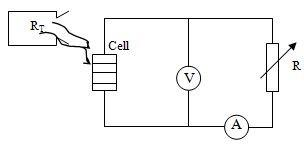Figure 2. Diagram of the solar cell

3. Material Used

The material used for the experimental part is:

1. A simulator of universal the Prado type using a lamp with halogen giving a spectrum almost identical to the sun.

2. An optical bench on which the cell is placed allowing to vary flow.

3. A rheostat allowing to vary the intensity and the voltage of the cell.

4. A ampermeter being used to measure the current of the cell.

5. A voltmeter being used to measure the voltage of the cell.

6. A sunshine recorder of the type Sharp Solar being used to measure flow.

7. A solar silicon cell.

4. Method of Determining the Parameter Extraction

Different techniques have been presented to extract the parameters of the two diode model without using least mean squares fitting .

When the model equation and its derivative are expressed at open circuit voltage and are short circuit, four independent equations are obtained. When normal cell parameters taken into account, these can be rewritten as in equations :

${{I}_{ph}}={{I}_{sc}}$   (5)

${{R}_{sh}}=\frac{1}{{{\left( \frac{\partial I}{\partial V} \right)}_{V=0}}}$   (6)

${{R}_{s}}=-\frac{1}{{{\left( \frac{\partial I}{\partial V} \right)}_{V={{V}_{\infty }}}}}-\frac{1}{\frac{{{I}_{01}}}{{{V}_{t}}}\exp \left( \frac{{{V}_{OC}}}{{{V}_{t}}} \right)+\frac{{{I}_{02}}}{2{{V}_{t}}}\exp \left( \frac{{{V}_{OC}}}{2{{V}_{t}}} \right)+\frac{1}{{{R}_{sh}}}}$   (7)

${{I}_{ph}}={{I}_{01}}\left( \exp \left( \frac{{{V}_{OC}}}{{{V}_{t}}} \right)-1 \right)+{{I}_{02}}\left( \exp \left( \frac{{{V}_{OC}}}{2{{V}_{t}}} \right)-1 \right)+\frac{{{V}_{oc}}}{{{R}_{sh}}}$   (8)

We developed a software allowing the determination of the parameters I01 defined in the equation characteristic of a solar cell.

Experimental determination of I01:

For various values (N) of flow, we determine I01 by using the following relations:

- For the model with exponential expression is following form :

${{I}_{01}}=\frac{1}{N}\sum\limits_{N}{\left[ \frac{{{I}_{cc}}N}{\left( \exp \left( w{{V}_{co}}N \right)-1 \right)} \right]}$   (9)

- For the model with two exponential we fix the current of I02 recombination, allowing calculates it current of I01 saturation which is given by the following expression :

${{I}_{01}}=\frac{{{I}_{cc}}N}{\left( \exp \left( w{{V}_{co}}N \right)-1 \right)}-\frac{{{I}_{02}}\left( \exp \left( w{{V}_{co}}_{N}/n \right)-1 \right)}{\left( \exp \left( w{{V}_{co}}_{N} \right)-1 \right)}-\frac{{{V}_{coN}}/{{R}_{sh}}}{\left( \exp \left( w{{V}_{co}}_{N} \right)-1 \right)}$   (10)

5. Results and Discussions

Our simulation program of characteristic I-V us allowed to obtain the variation of the parameters of the solar cell for various flows.

Table 1. Variation of the parameters of the I = f(V) characteristic according to flow

 f (W/m2) PM h (%) FFexp hexp (%) 400 0,202 11,49 0,691 9,92 550 0,287 11,87 0,679 10,01 700 0,369 11,96 0,680 10,07 850 0,459 12,30 0,711 10,80 1000 0,555 12,61 0,709 11,02

Indeed we notice that the factor of form and the efficiency increase with incidental flow. With an aim of comparing the two types of model, we simulated the characteristic of the solar cell for the value of flow f = 1000 W/m2 and the results of our simulation are given by the following figures.

## f3.pngFigure 3. Characteristic current-voltage of the model to exponential for various values of n and in f = 1000 W/m2

## f4.png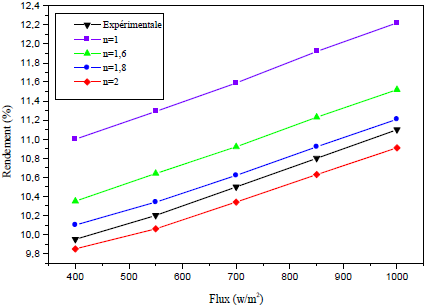Figure 4. Variation of the efficiency according to solar flow (I01 = 10-10 A)

5.1 Single exponential model

Experimental measurements are represented graphically in (*), according to the Figure 3, we notice that for a factor of quality equal to the unit a high efficiency was obtained but its value is not compatible with the experimental, while n = 1,8 the theoretical curve are almost confused compared to the experimental results.

The Figure 4 shows the variation of the output according to flow with current a diffusion I01 = 10-10 A of better results were obtained but with a factor of quality higher than one. So a search for new structure must relate to the improvement of factor of quality and the diffusion current. This shows the interest to use the model with two exponential in order to being able to differentiate the two phenomena, the diffusion and the recombination in zone of space charge in the simulation of the operation of the solar cell according to the parameters of the characteristic voltage.

5.2 Double exponential model

Figures 5, 6 and 7 show us the impact of the parameters of the model to two exponential on the characteristic current-tension of a cell. We note that for a significant value of the current of recombination, high outputs can be obtained, we notice that the value nearest with experimental is between 10-5 and 1.5×10-5 A/cm2. We also note that for a factor of quality N given, the influence of the current of I02 recombination is dominating in front of that of current I01 the diffusion. And for a great value of the factor of quality of the diode (n=2,2), the theoretical curve is similar to the experimental with a cell with a significant current of recombination and a weak diffusion current of about 10-10 A/cm2.

## f5.png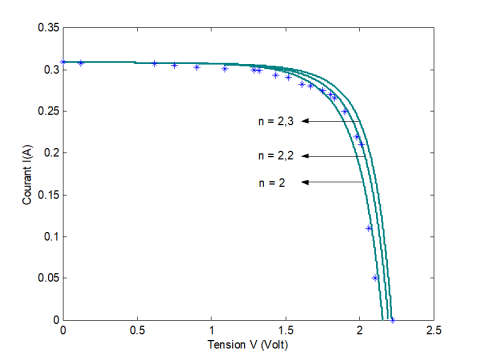Figure 5. Influence factor of quality on the model with two exponential

## f6.pngFigure 6. Influence of diffusion current on the model with two exponential

## f7.png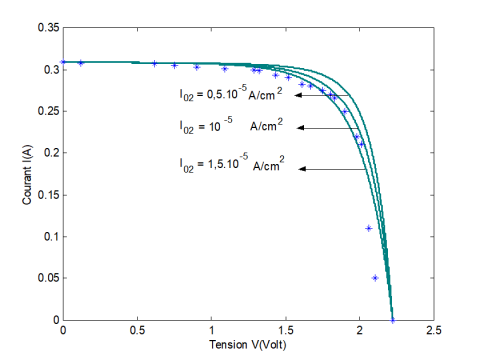Figure 7. Influence of I02 on the model with two exponential

## f8.png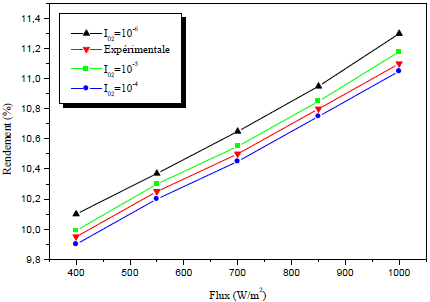Figure 8. Variation of the output according to solar flow (n=2,2)

According to the Figure 8 we deduce that for a current from recombination I02 = 10-5 A/cm2, for a factor of quality higher than 2; the theoretical yields and experimental are close. This result informs us about the importance of the type and the position of the levels traps in the determination of the output h since the maximum of the current of recombination in zone of space charge arises for levels of traps located at the medium of the forbidden band (n=2).

6. Conclusions

Our study with carried on the analysis of the two models allowing the determination of the parameters of a photovoltaic cell. The model with two exponential allowing to investigate the phenomena of recombination in zone of diffusion and space charge in the areas quasi-neutrals of the transmitter and the base.

Our experimental results were compared to the theory for the two models describing the operation of the silicon solar cell.

This study underlines the insufficiency of the model to exponential generally used by showing that it leads to the design of the ideal solar cells whereas structures characterized by a factor of quality greater outcome with high efficiency.

As a perspective of this work, we plan to carry out additional experimental studies for photovoltaic modules based on polycrystalline and amorphous silicon; and to develop the model with resolution methods to determine different specific parameters of the current-voltage characteristic.

References

 Zerga, A. (1998). Optimisation du rendement d’une cellule solaire à base de silicium monocristallin de type n+p, Thèse de Magister, Université de Tlemcen.

 Enebish, N., Agachbayar, D., Dorjkhand, S., Baatar, D., Ulemj, I. (1993). Numerical analysis of solar cells current-voltage characteristic. Solar Energy Materials and Solar Cells, 29: 201-208. https://doi.org/10.1016/0927-0248(93)90035-2

 Lakeh, H.K., Kaatuzian, H., Hosseini, R. (2019). A parametrical study on photo-electro-thermal performance of an integrated thermoelectric-photovoltaic cell. Renewable Energy, 138: 542-550. https://doi.org/10.1016/j.renene.2019.01.094

 Jeong, Y., Shin, D., Park, J.H., Park, J., Yi, Y., Im, S. (2019). Integrated advantages from perovskite photovoltaic cell and 2D MoTe2 transistor towards self-power energy harvesting and photosensing. Nano Energy, 63: 103833. https://doi.org/10.1016/j.nanoen.2019.06.029

 Antonacci, A., Scognamiglio, V. (2019). Photosynthesis-based hybrid nanostructures: electrochemical sensors and photovoltaic cells as case studies. TrAC Trends in Analytical Chemistry, 115: 100-109. https://doi.org/10.1016/j.trac.2019.04.001

 Gruber, M., Jovanov, V., Wagner, V. (2019). Modeling of photoactive area spreading in unstructured photovoltaic cells. Solar Energy Materials and Solar Cells, 200: 110011. https://doi.org/10.1016/j.solmat.2019.110011

 Katayama, N., Osawa, S., Matsumoto, S., Nakano, T., Sugiyama, M. (2019). Degradation and fault diagnosis of photovoltaic cells using impedance spectroscopy. Solar Energy Materials and Solar Cells, 194: 130-136. https://doi.org/10.1016/j.solmat.2019.01.040

 Hernández-Callejo, L., Gallardo-Saavedra, S., Alonso-Gómez, V. (2019). A review of photovoltaic systems: Design, operation and maintenance. Solar Energy, 188: 426-440. https://doi.org/10.1016/j.solener.2019.06.017

 Quan, L., Xie, K., Liu, Y., Zhang, H. (2019). Camera enhanced compressive light beam induced current sensing for efficient defect detection in photovoltaic cells. Solar Energy, 183: 212-217. https://doi.org/10.1016/j.solener.2019.02.055

 Alcañiz, A., López, G., Martín, I., Jiménez, A., Datas, A., Calle, E., Rosa, E., Gerling, L.G., Voz, C., del Cañizo, C., Alcubilla, R. (2019). Germanium photovoltaic cells with MoOx hole-selective contacts. Solar Energy, 181: 357-360. https://doi.org/110.1016/j.solener.2019.02.009

 Shittu, S., Li, G., Akhlaghi, Y.G., Ma, X., Zhao, X., Ayodele, E. (2019). Advancements in thermoelectric generators for enhanced hybrid photovoltaic system performance. 109: 24-54. https://doi.org/10.1016/j.rser.2019.04.023

 Salem, M.R., Elsayed, M.M., Abd-Elaziz, A.A., Elshazly, K.M. (2019). Performance enhancement of the photovoltaic cells using Al2O3/PCM mixture and/or water cooling-techniques. Renewable Energy, 138: 876-890. https://doi.org/10.1016/j.renene.2019.02.032

 Torabi, N., Behjat, A., Zhou, Y., Docampo, P., Stoddard, R.J. (2019). Hillhouse H.W., Ameri T., Progress and challenges in perovskite photovoltaics from single- to multi-junction cells. Materials Today Energy, 12: 70-94. https://doi.org/10.1016/j.mtener.2018.12.009

 Vargas-Estevez, C., Blanquer, A., Murillo, G., Duque, M., Barrios, L., Nogués, C., Ibañez, E., Esteve, J. (2018). Electrical stimulation of cells through photovoltaic microcell arrays. Nano Energy, 51: 571-578. https://doi.org/10.1016/j.nanoen.2018.07.012

 Mathews, I., Kantareddy, S.N., Buonassisi, T., Peters, I.M. (2019). Technology and market perspective for indoor photovoltaic cells. Joule, 3(6): 1415-1426. https://doi.org/10.1016/j.joule.2019.03.026

 Shaygan, M., Ehyaei, M.A., Ahmadi, A., El Haj Assad, M., Silveira, J.L. (2019). Energy, exergy, advanced exergy and economic analyses of hybrid polymer electrolyte membrane (PEM) fuel cell and photovoltaic cells to produce hydrogen and electricity. Journal of Cleaner Production, 234: 1082-1093. https://doi.org/10.1016/j.jclepro.2019.06.298

 Falama, R.Z., Hidayatullah, Doka, S.Y. (2019). A promising concept to push efficiency of pn-junction photovoltaic solar cell beyond Shockley and Queisser limit based on impact ionization due to high electric field. Optik, 187: 39-48. https://doi.org/10.1016/j.ijleo.2019.04.136

 Ansari, Z.A., Singh, T.J., Islam, S.M., Singh, S., Mahala, P., Khan, A., Singh, K.J. (2019). Photovoltaic solar cells based on Graphene/Gallium arsenide schottky junction. Optik, 182: 500-506. https://doi.org/10.1016/j.ijleo.2019.01.078

 Cotfas, D.T., Deaconu, A.M., Cotfas, P.A. (2019). Application of successive discretization algorithm for determining photovoltaic cells parameters. Energy Conversion and Management, 196: 545-556. https://doi.org/10.1016/j.enconman.2019.06.037

 Aguiar, A., Farinhas, J, da Silva, W., Ghica, M.E., Brett, C.M.A., Morgado, J., Sobral, A.J.F.N. (2019). Synthesis, characterization and application of meso-substituted fluorinated boron dipyrromethenes (BODIPYs) with different styryl groups in organic photovoltaic cells. Dyes and Pigments, 168: 103-110. https://doi.org/10.1016/j.dyepig.2019.04.031

 Manfredi, N., Trifiletti, V., Melchiorre, F., Giannotta, G., Biagini, P., Abbotto, A. (2019). Photovoltaic characterization of di-branched organic sensitizers for DSSCs. Data in Brief, 2019. https://doi.org/10.1016/j.dib.2019.104167

 Charles, J.P., Mekkaoui, I., Bordure, G. (1985). A critical study of the effectiveness of the single and double exponential models for I-V characterization of solar cell. Solid-State Electronics, 28: 807-820. https://doi.org/10.1016/0038-1101(85)90068-1

 Sari-Ali, I. (2003). Contribution à l’etude de la caractéristique courant-tension des cellules solaires fonctionnant sous eclairement et a l’obscurité. Thèse de Magister, Université de Tlemcen.